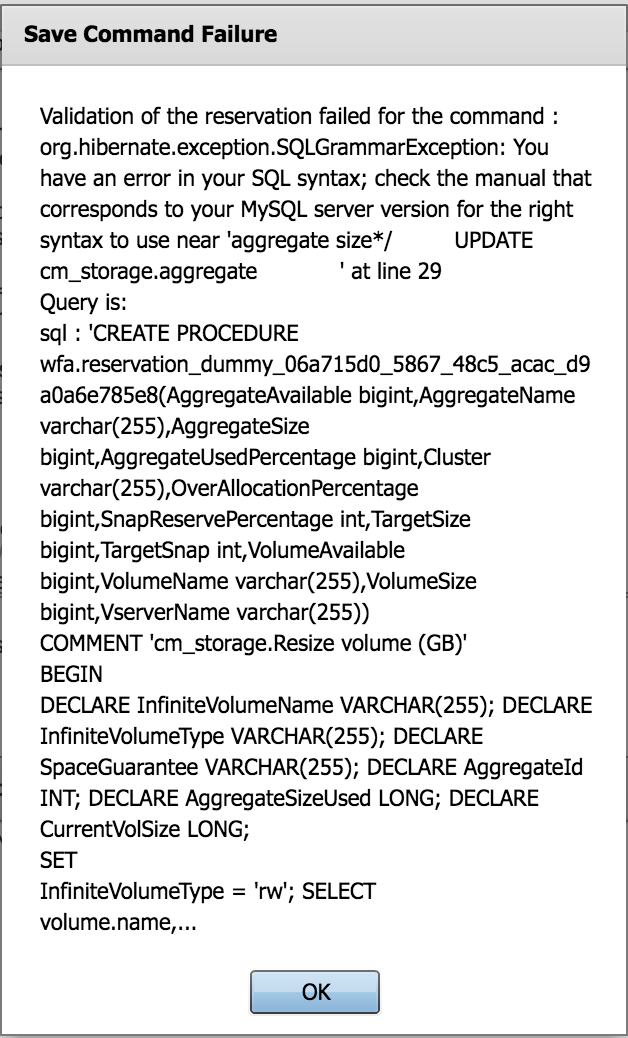Active IQ Unified Manager Discussions

## WFA 4.2 (on linux) Eating Line Endings1,132 Views

So we have recently discovered an issue after upgrading from WFA 4.0 to 4.2 where line endings are getting removed.  We have noticed this in other areas of WFA 4.2, but this is the best example that demonstrates the issue.

Here is a small bit SQL from the reservation for the Netapp supported "cm_storage" -  "Resize Volume" .

```...IF InfiniteVolumeType = 'rw' THEN
-- First add this size to the size of the overall infinite volume
UPDATE
cm_storage.volume infinite_vol
JOIN
cm_storage.vserver vserver
ON vserver.id = infinite_vol.vserver_id
AND vserver.name = '\${VserverName}'
JOIN
cm_storage.cluster cluster
ON cluster.id = vserver.cluster_id
AND (
cluster.name = '\${Cluster}'
)
AND infinite_vol.is_managed_by_service IS TRUE
AND infinite_vol.style = 'infinivol'
SET
infinite_vol.size_mb = (infinite_vol.size_mb + AggregateSizeUsed );
END IF;...```

In 4.0 when you hit "save" on this command you would get a success, as you would expect.

In 4.2 you now receive this error...The way we fixed this was either remove the single line comment or convert it to a multiline comment...

```IF InfiniteVolumeType = 'rw' THEN
/* First add this size to the size of the overall infinite volume */
UPDATE
cm_storage.volume infinite_vol
JOIN
cm_storage.vserver vserver
ON vserver.id = infinite_vol.vserver_id
AND vserver.name = '\${VserverName}'
JOIN
cm_storage.cluster cluster
ON cluster.id = vserver.cluster_id
AND (
cluster.name = '\${Cluster}'
)
AND infinite_vol.is_managed_by_service IS TRUE
AND infinite_vol.style = 'infinivol'
SET
infinite_vol.size_mb = (infinite_vol.size_mb + AggregateSizeUsed );
END IF; ```

Another odd thing is that if you look at the SQL in WFA 4.0 it looks like nicely formatted SQL

```DECLARE InfiniteVolumeName VARCHAR(255);
DECLARE InfiniteVolumeType VARCHAR(255);
DECLARE SpaceGuarantee VARCHAR(255);
DECLARE AggregateId INT;
DECLARE AggregateSizeUsed LONG;
DECLARE CurrentVolSize LONG;```

Here is how it looks in 4.2

`DECLARE InfiniteVolumeName VARCHAR(255); DECLARE InfiniteVolumeType VARCHAR(255); DECLARE SpaceGuarantee VARCHAR(255); DECLARE AggregateId INT; DECLARE AggregateSizeUsed LONG; DECLARE CurrentVolSize LONG; `

It seems that the "\n" is getting removed and I assume it is happening in WFA and not in the mysql part.

Our workaround of changing the comments to multiline comments is working, but this is something that needs to be addressed in the next version of WFA (linux)

0 REPLIES 0